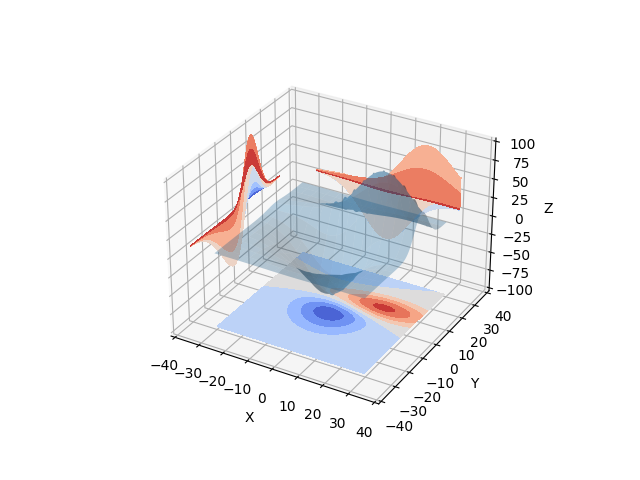Projecting filled contour onto a graph¶

Demonstrates displaying a 3D surface while also projecting filled contour 'profiles' onto the 'walls' of the graph.

See contour3d_demo2 for the unfilled version.from mpl_toolkits.mplot3d import axes3d
import matplotlib.pyplot as plt
from matplotlib import cm

X, Y, Z = axes3d.get_test_data(0.05)

# Plot the 3D surface
ax.plot_surface(X, Y, Z, rstride=8, cstride=8, alpha=0.3)

# Plot projections of the contours for each dimension.  By choosing offsets
# that match the appropriate axes limits, the projected contours will sit on
# the 'walls' of the graph
cset = ax.contourf(X, Y, Z, zdir='z', offset=-100, cmap=cm.coolwarm)
cset = ax.contourf(X, Y, Z, zdir='x', offset=-40, cmap=cm.coolwarm)
cset = ax.contourf(X, Y, Z, zdir='y', offset=40, cmap=cm.coolwarm)

ax.set_xlim(-40, 40)
ax.set_ylim(-40, 40)
ax.set_zlim(-100, 100)

ax.set_xlabel('X')
ax.set_ylabel('Y')
ax.set_zlabel('Z')

plt.show()

Keywords: matplotlib code example, codex, python plot, pyplot Gallery generated by Sphinx-Gallery